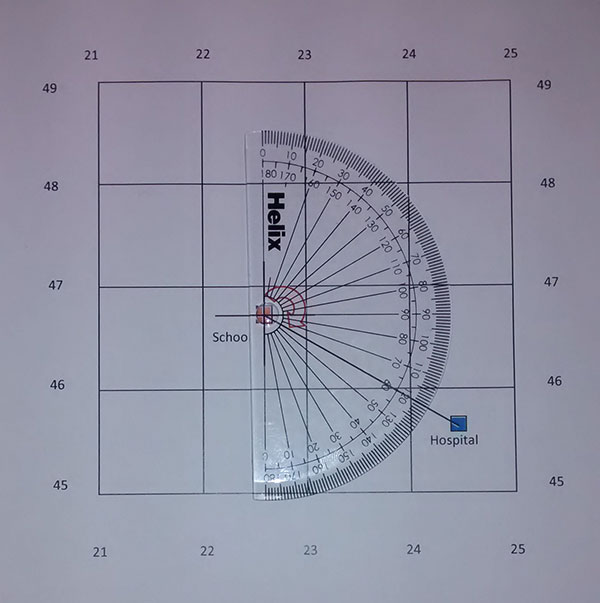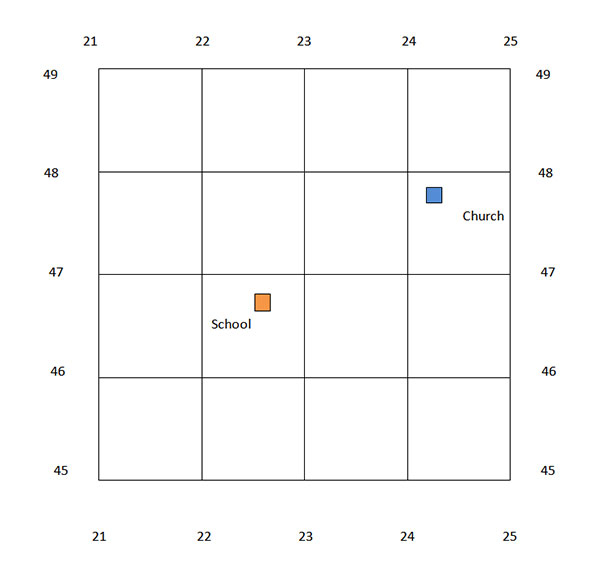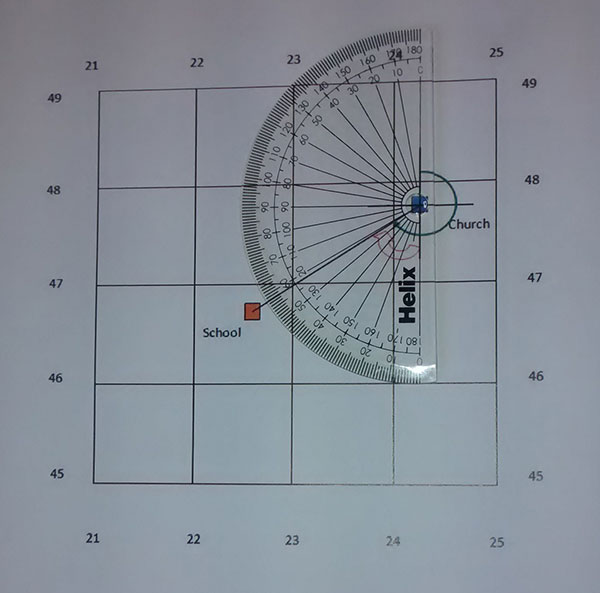Select Page

# Compass Bearing

The CXC Geography syllabus requires students to be able to give directions in terms of compass bearing. When giving a bearing, direction is indicated using angles. North is indicated as 0 degrees. Angles are measured in a clockwise direction from north (see below).On this compass you can see that the angles are measured in a clockwise direction from north. (Photo by Bios)

Looking at the compass above you can see that 0 degrees represents north, 90 degrees represents east, 180 degrees represents south and 270 degrees represents west. The direction of one place from another can be given by measuring the exact angle from north.

## Giving a Bearing

When giving a bearing it is important to figure out your starting point and end point. The question may be posed like this:

Give the bearing from A to B. (in this case your starting point is A and your end point is B)

Or the question may be posed like this:

Give the bearing of A from B. (In this case your starting point is B and your end point is A)

Note: The word “from” lets you know where to start.

When you have figured out your starting point, the next step is to draw a cross right on top of it. The cross consists of a vertical line and a horizontal line. These lines should intersect exactly on your starting point. Next, draw a line from the middle of the cross to your end point.

Place a protractor over the cross. Ensure that the 90 degree line on your protractor runs along your horizontal line and the line representing 0 and 180 degrees runs along your vertical line. The point where these two lines intersect should be right in the middle of the cross. Your protractor must be properly placed in order for you to get an accurate measurement. Then you simply measure the size of the angle between north and the line drawn to the end point, moving clockwise.

Example 1:

Look at the grid below. What is the bearing of the hospital in 2445 from the school in 2246?The first step is to figure out the start and end points. Since we’re going from the school, our starting point is the school. Our end point is the hospital. Next we draw a cross directly over the school. Then we draw a line from the middle of the cross to the middle of the hospital (see below).Then we simply measure the angle going clockwise from 0 degrees (north) to the line which goes to the hospital. In the picture above this angle is indicated by the red arrow. The picture below shows the correct placement of the protractor for measuring the angle.Notice that the line representing 0 and 180 degrees runs along the vertical line and the 90 degree line runs along the horizontal line toward the east. Can you tell the size of the angle?

The size of the angle is 120 degrees. Therefore we can say that the bearing of the hospital from the school is 120 degrees.

Example 2:

You may be asked to give the bearing of an object located somewhere to the western side of your starting point. In that case, the procedure will be slightly different. Consider this question:

What is the bearing of the school in 2246 from the church in 2447?Since we’re going from the church, our starting point is the church and our end point is the school. We draw a cross right on top of the church. Then we draw a line from the middle of this cross to the school. This has been done below.Now we need to measure the angle from north to the line which goes to the school. In the picture above, this angle is indicated by the green arrow. However, we do not need to measure this entire angle. We already know that the angle from north to south is 180 degrees. All we need to do is measure the rest of the angle (indicated by the red arrow) and add 180 degrees to it. First, we place the protractor as shown in the picture below.Notice that the line representing 0 and 180 degrees runs along the vertical line and the 90 degree line runs along the horizontal line toward the west. Can you tell the size of the angle?

Next we measure the size of the angle in a clockwise direction from south to the line going to the school. The size of this angle is 58 degrees. However, this is not our final answer. Remember we are measuring the angle in a clockwise direction from north. Also remember that the angle from north to south is 180 degrees. Therefore our final answer is determined as follows: 180 degrees + 58 degrees = 238 degrees. Therefore we can say that the bearing of the school from the hospital is 238 degrees.

References

http://commons.wikimedia.org/wiki/File:Kompas_Sofia.JPG

http://geology.isu.edu/geostac/Field_Exercise/topomaps/bearing.htm

### Related Pages

Map Skills

Giving Directions

Four Figure Grid References